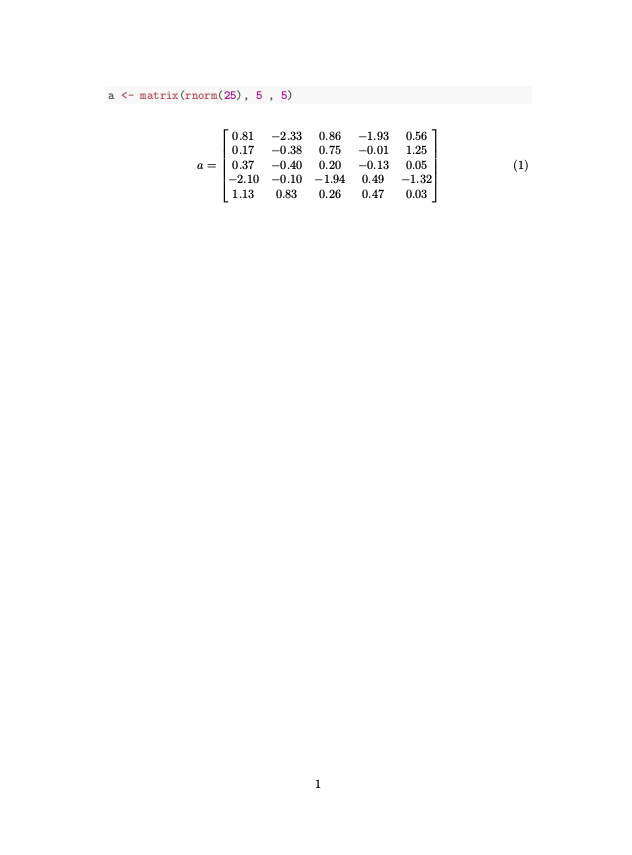Brett Klamer

# Print R Matrices in LaTeX Math Environments

This is a quick and easy method to print R matrices in LaTeX math environments. It’s based on the R function found here. (The newline at the end of the bmatrix should be removed)

Use this function

bmatrix = function(x, digits=NULL, ...) {
library(xtable)
default_args = list(include.colnames=FALSE, only.contents=TRUE,
include.rownames=FALSE, hline.after=NULL, comment=FALSE,
print.results=FALSE)
passed_args = list(...)
calling_args = c(list(x=xtable(x, digits=digits)),
c(passed_args,
default_args[setdiff(names(default_args), names(passed_args))]))
return(cat("\\begin{bmatrix}\n",
do.call(print.xtable, calling_args),
"\\end{bmatrix}"))
}


And then insert the matrix as seen here

\begin{equation}
a =
<<results = 'asis', echo=FALSE>>=
bmatrix(a)
@
\end{equation}


Grab the .Rnw example file here. It should compile into something like thisPublished: 2014-09-02
Last Updated: 2014-09-09